+
Using z-Scores to find a Probability
Interpret Inferential Statistics
0
of 0 possible points
1 Tutorials that teach Using z-Scores to find a Probability

# Using z-Scores to find a Probability

Author: Sophia Tutorial
##### Description:

Provided with a table learner can find proportions based on z-scores.

(more)

Sophia’s self-paced online courses are a great way to save time and money as you earn credits eligible for transfer to many different colleges and universities.*

No credit card required

37 Sophia partners guarantee credit transfer.

299 Institutions have accepted or given pre-approval for credit transfer.

* The American Council on Education's College Credit Recommendation Service (ACE Credit®) has evaluated and recommended college credit for 32 of Sophia’s online courses. Many different colleges and universities consider ACE CREDIT recommendations in determining the applicability to their course and degree programs.

Tutorial
what's covered
This lesson discusses using z-scores to find a probability. By the end of this lesson, you will be able to use a z-score probability table to determine the likelihood of a range of values of a normally distributed variable occurring. This lesson covers:
1. Normal Distribution and z-Scores
2. z-Tables
3. How z-Tables Are Used

## 1. Normal Distribution and z-Scores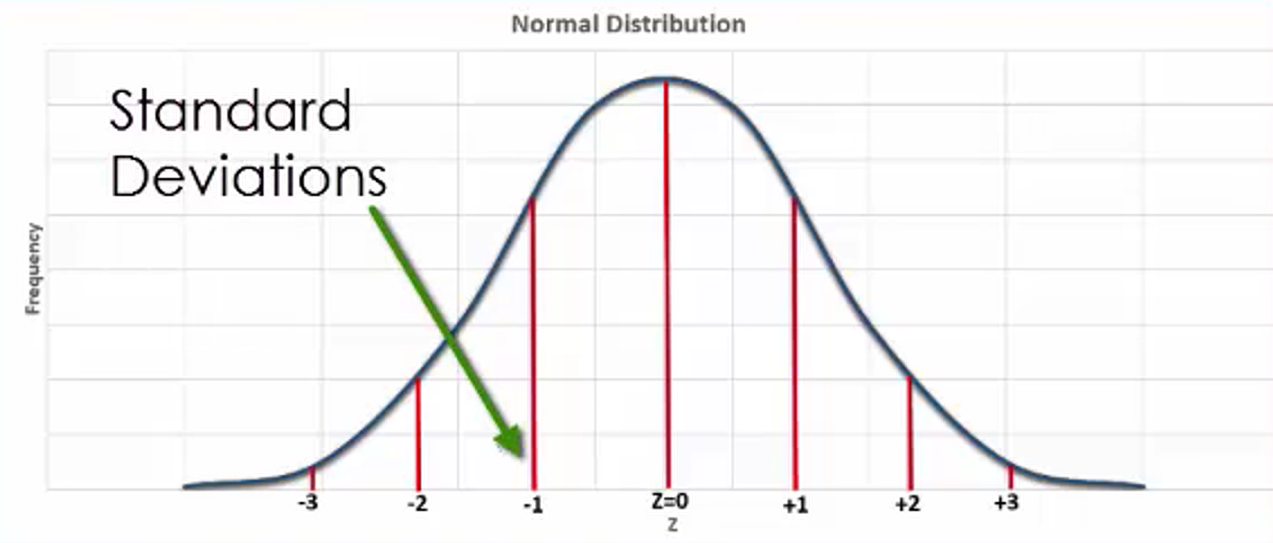The graph you see here is that of a standard, normally distributed variable, otherwise known as a z-distribution. Because they allow you to know the position of a value in a normal distribution, z-scores are important. When you know a z-score associated with the value of a variable, you are able to determine the likelihood that an event will take place. Simply having a solid understanding of z-scores and how they are associated to probability allows you to easily determine the likelihood of an event occurring.

Approximately 68% of values in a normally distributed variable fall between a z-score of -1 and 1. 95% of values fall between a value of -2 and 2. 99.7% of values fall between a z-score of -3 and 3. If you were to think of likely values in a normal distribution, they would fall in the 95% between a z-score of -2 and 2.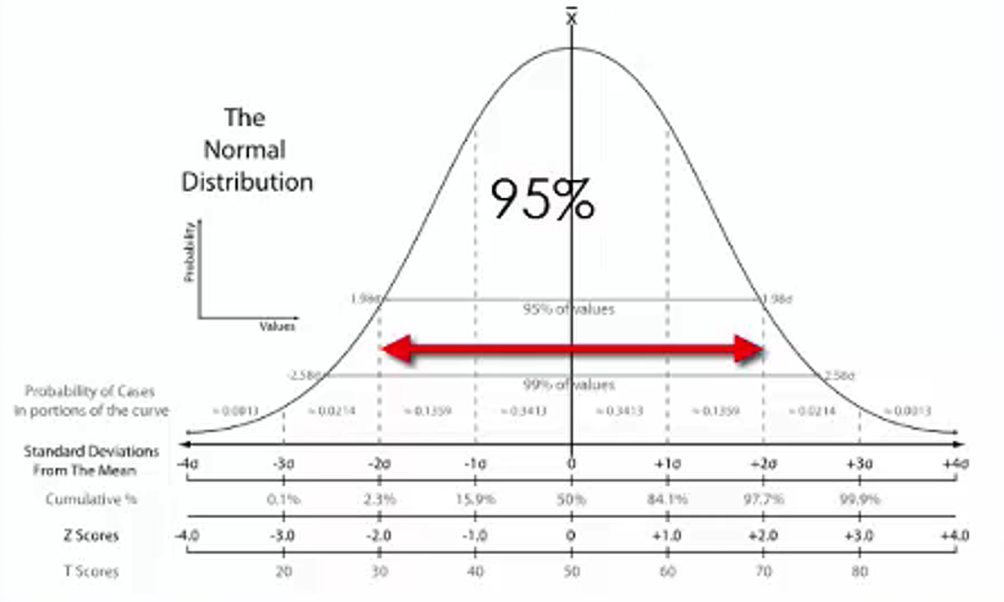If a value has a large positive z-score, you would consider it to be unusually large, and not very likely to occur. Along the same line of thought, if a value has a large negative z-score, you would consider it to be unusually small, and not very likely to occur either.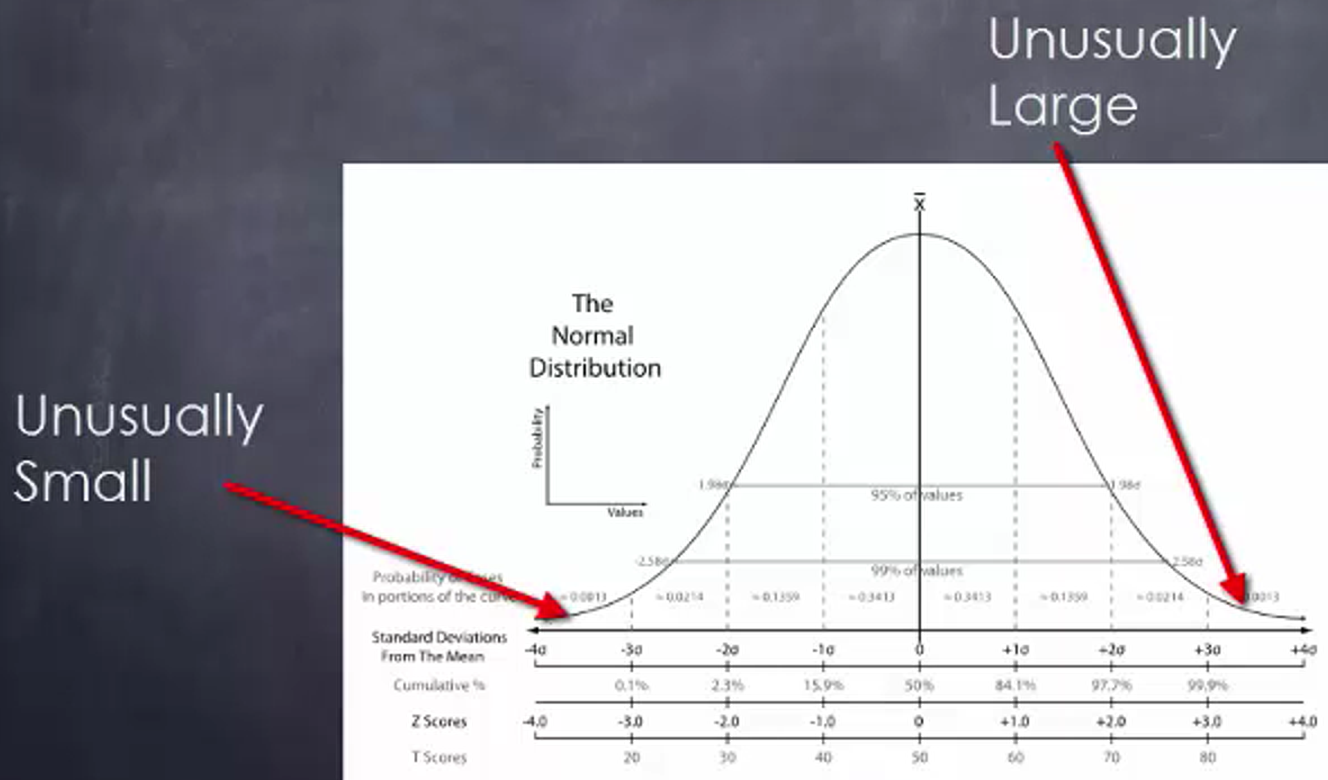Suppose you were to take a look at the mean area of all homes in the United States in terms of square feet. Say that the mean area of a home in the United States is 2,400 square feet, with a standard deviation of 400 square feet. If you had a home that has 1,450 square feet, the z-score would be equal to -2.375.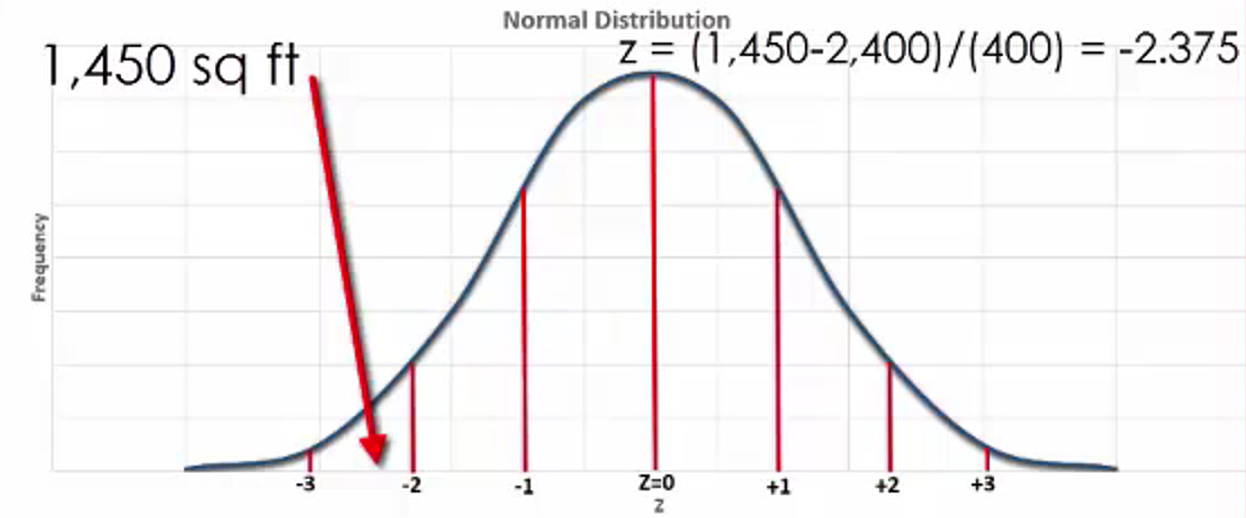If there was a home with an area of 2,300 square feet, the z-score would be equal to -0.25 using the same z-score formula.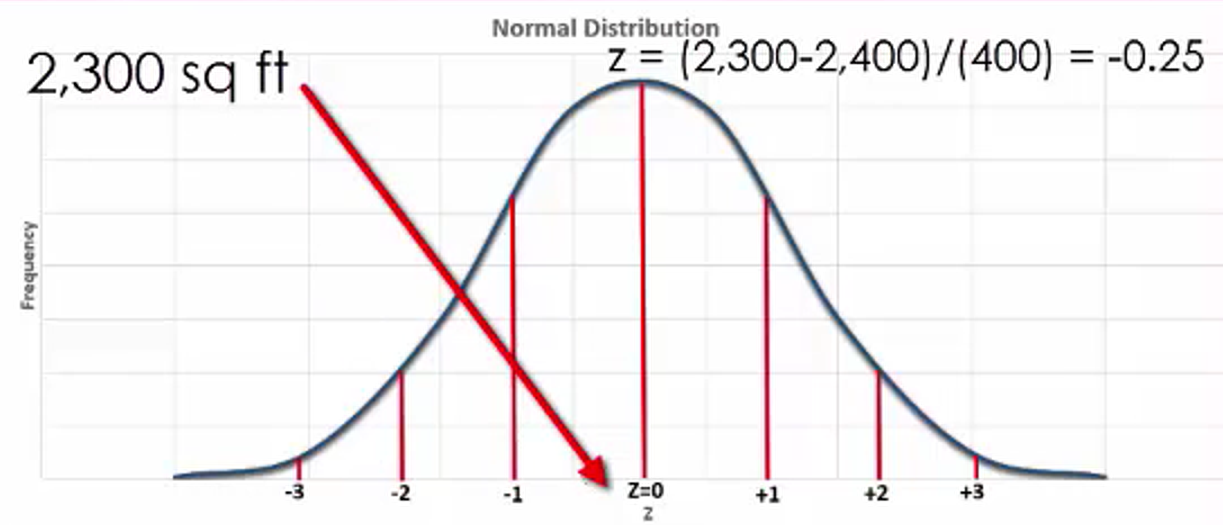If there was a home with an area 4,080 square feet, the z-score would be equal to 4.2 based upon the same calculations.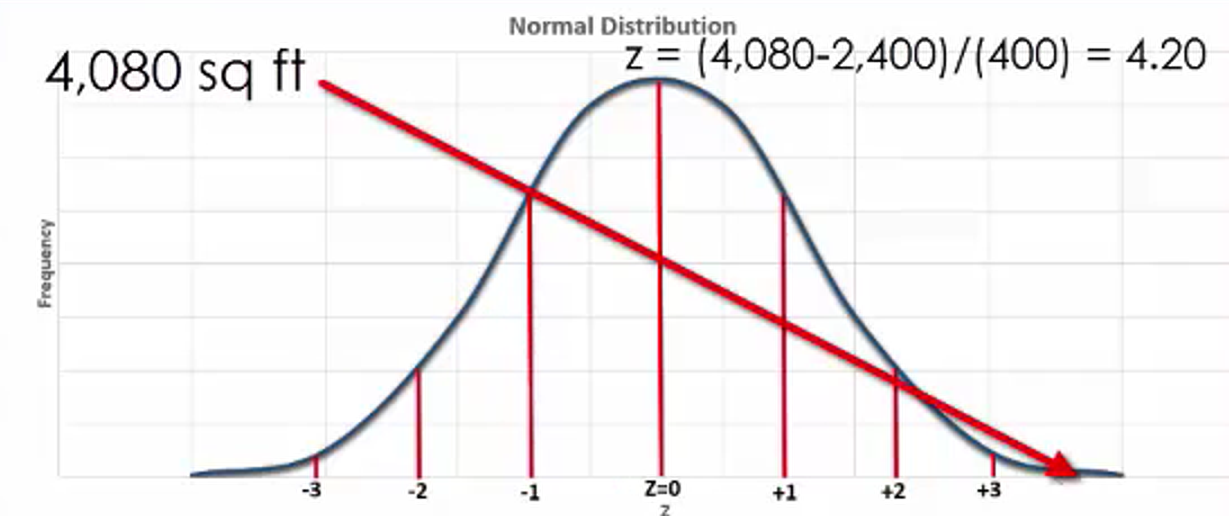Of these three z-scores, which are likely to occur?

The 2,300-square-foot home is likely to occur in a sample of homes drawn from a normally distributed population. However, the 1,450-square-foot home and the 4,080-foot-square home would be very unlikely because they don’t fall between a z-score of -2 and 2.

## 2. z-Tables

Since the z-distribution is a standard normal distribution, z-scores and probabilities that are associated with a specific z-score can be represented on standard tables. This being the case, you can use a table to find the areas under a z-distribution curve.

When viewing a normal distribution graph, the area under the curve is associated with the probabilities of a value occurring. So knowing the area corresponding to a z-score tells us about the probability of an event taking place.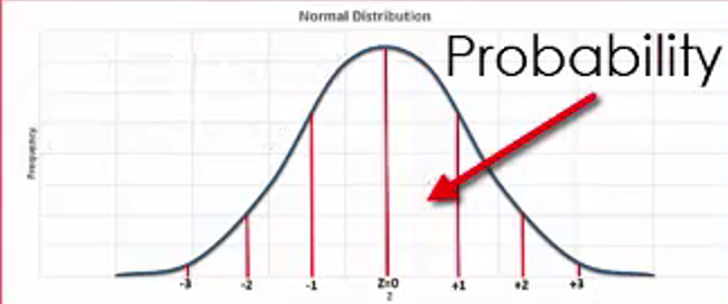As you can see, the positive z-table begins at the center of the z-distribution, where z is equal to 0 and represents the mean of the distribution. The negative z-table, on the other hand, starts out at the left of the distribution, and shows increasing z values up to the point of z being equal to 0.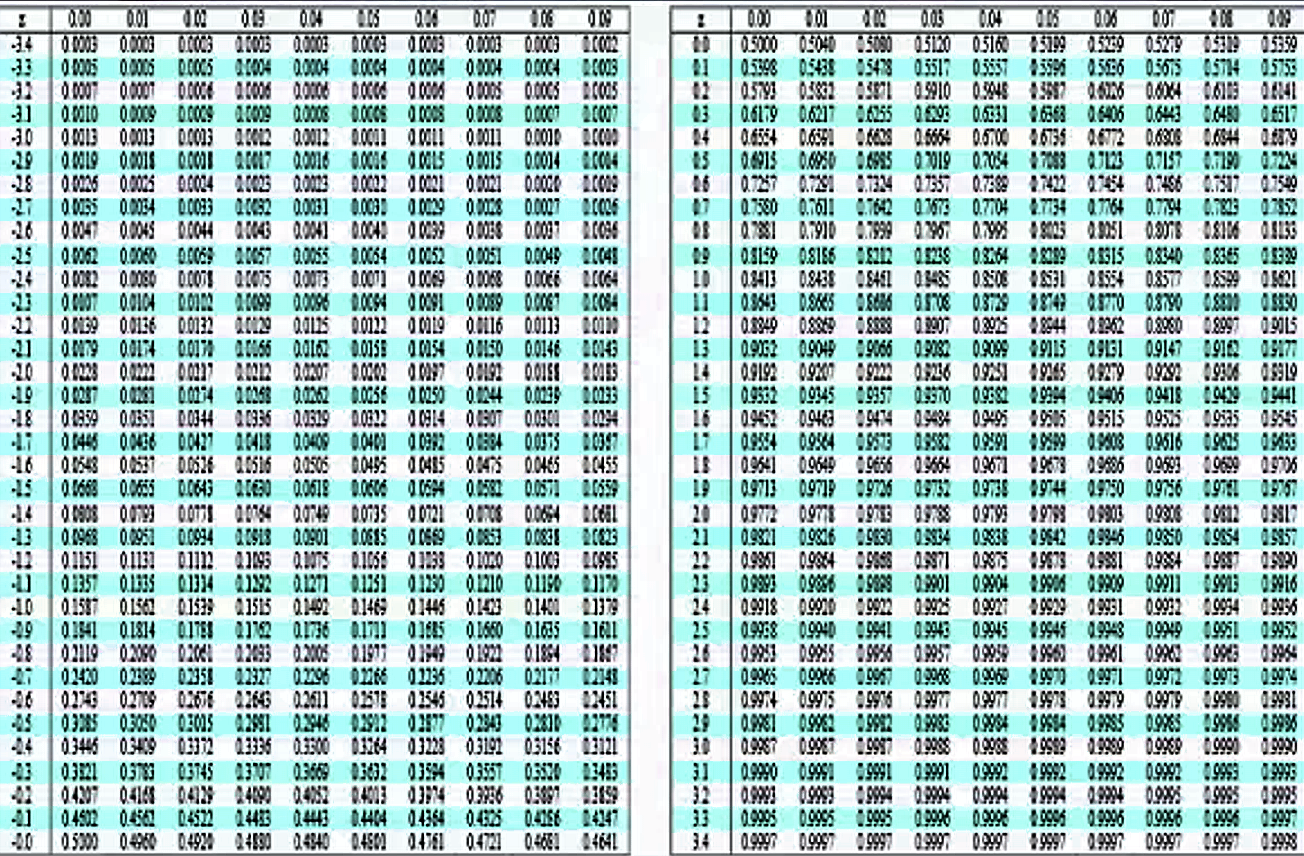The values for both z-tables correspond with the same information that is illustrated in a z-distribution graph, but actually tell more about the specific likelihood of an event occurring.

If you are provided with a negative z-score, you use the negative z-table to find the area under the curve to the left of the given z-score. This area under the curve is equal to the proportion of all z-scores in this range. When you have a positive z-score, the positive z-table lets you find the area under the curve to the left of that given z-score. This area under the curve is equal to the proportion of all z-scores in this range.

term to know
z-Table
Table for looking up the area starting at z = 0 for a positive z-score

## 3. How z-Tables Are Used

Take a look at ACT scores to illustrate this. The American College Test is taken by high school students all across the United States as a means of determining their aptitude for attending college. Let’s suppose in this instance that the mean score for the population is 21, and the standard deviation in this case is 5. How will you determine the probability that a score would fall within a particular range?

Consider the probability that the score would be higher than 30. How do you determine what that value is? The first thing you do is use the z-score formula to figure out what the z-score is. In this case, it is the difference between 30 and 21, which is 9, divided by the standard deviation of 5, which gives you a z-score of 1.8. If you look at the z-table below, that gives you a probability value of 0.9641.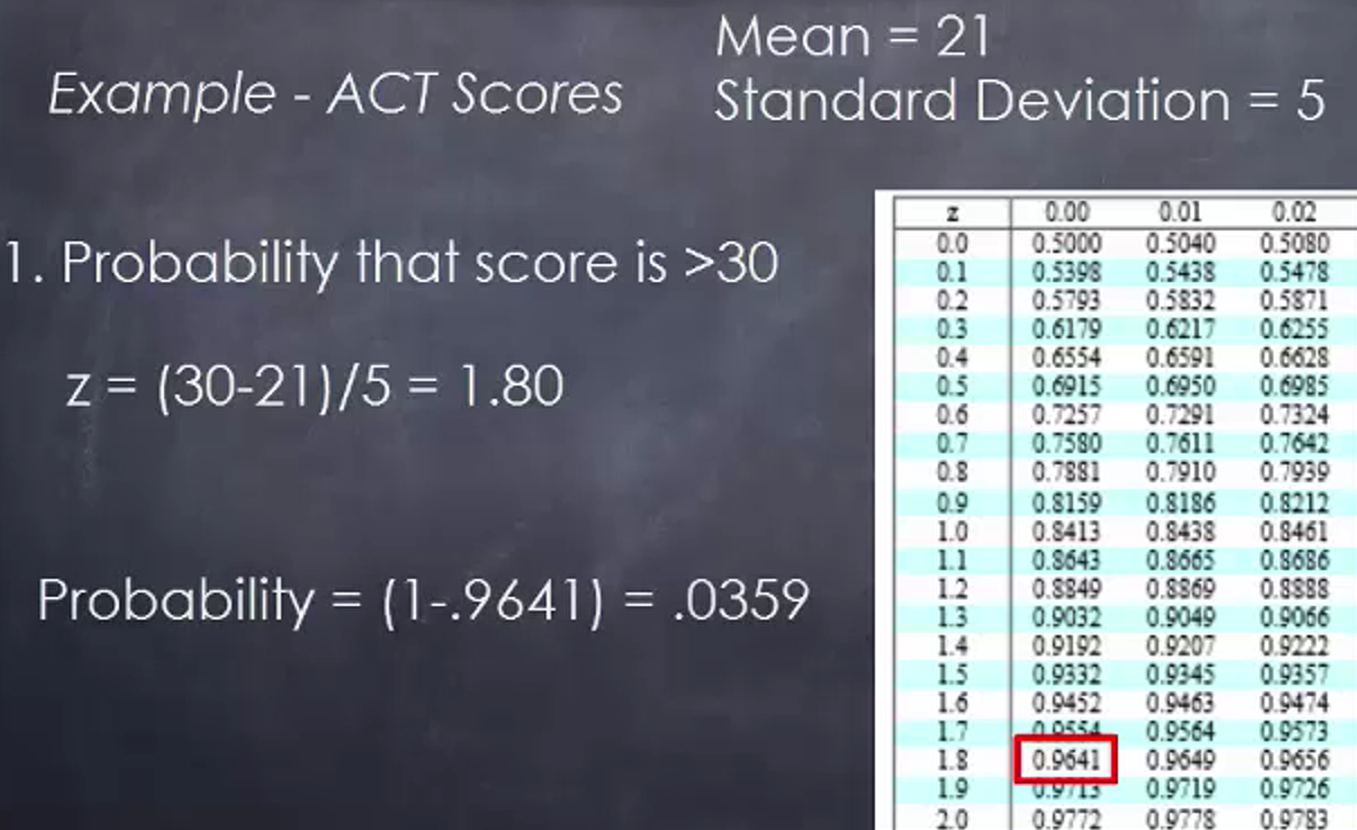Now, what does that tell you? It tells you that the area to the left of that point is 0.9641, or roughly 96% of the area under the curve falls to the left of z equal to 1.8. To determine the probability that the score is greater than 30, you’re interested in the difference between 0.9641 and 1, which gives you a probability of 0.0359.

What if you’re interested in the probability that a score falls between 23 and 27? In this instance, you need to calculate two different z-scores, one for 23 and one for 27. Locate both of these z-scores on the z-table. Then subtract the difference between the greater value and the lower value. That is the probability that a randomly drawn score would fall between the range of 23 and 27.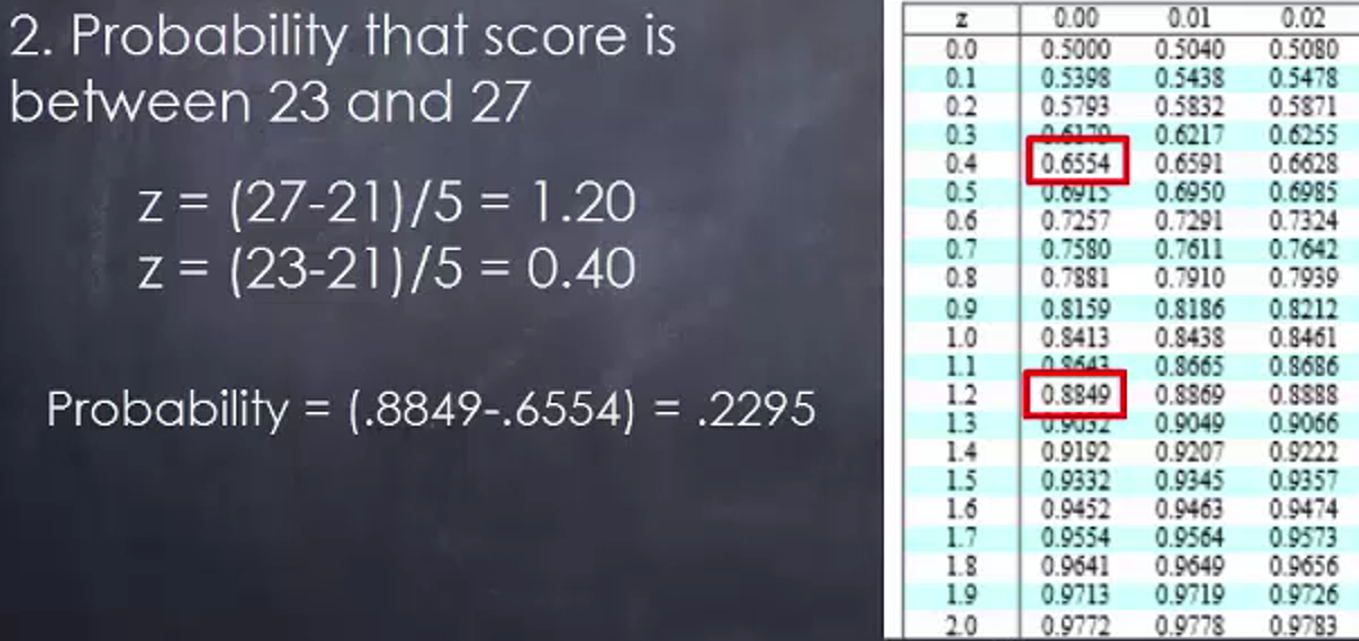What if you’re looking at some areas to the left of the mean? Suppose you’re interested in the probability that a score falls between 15 and 20. You will run the same type of calculations. The z-score for a 20 would be equal to a negative 0.2. The z-score for 15 would be equal to negative 1.2. Once again, you figure out the probabilities associated with each value by finding the difference. This works out to be a probability of 0.3056.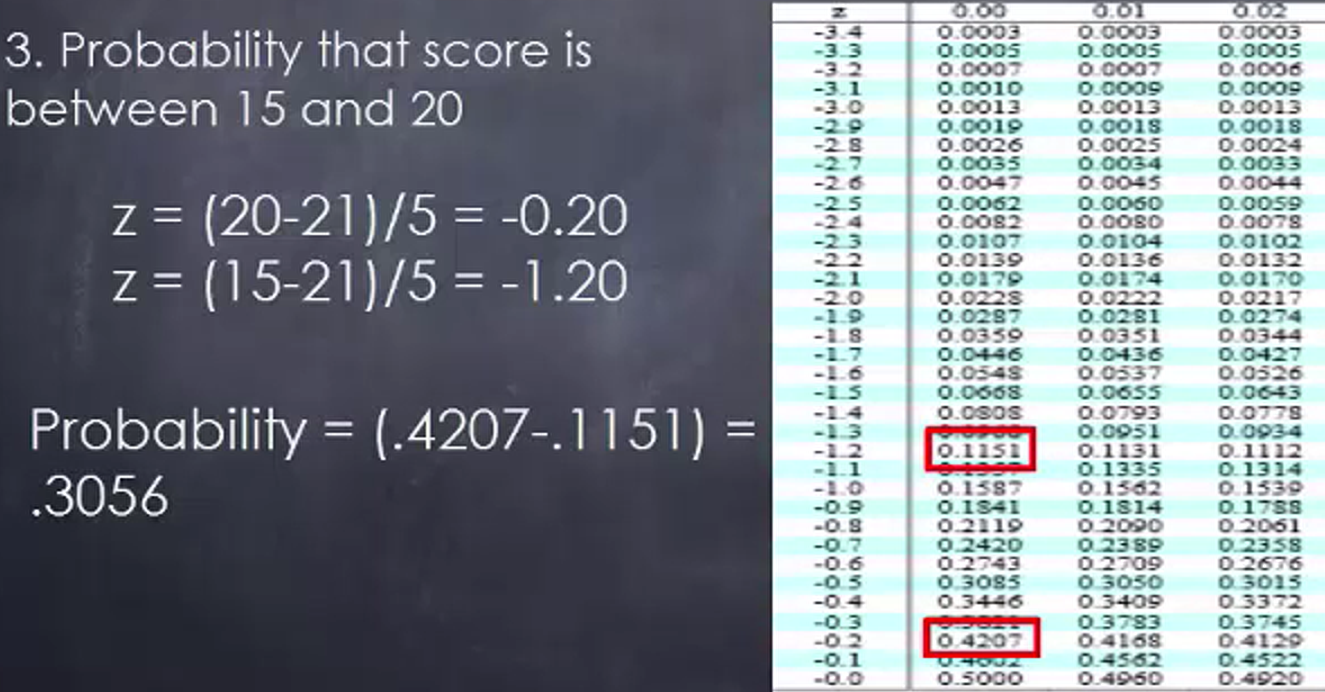Lastly, look at the probability that a score will be less than 20. Here you simply need to find the z-score for 20, which is equal to -0.20. The probability for this value is 0.4207, which tells you that’s the probability that a score would be below 20, based upon a normal distribution.What if you’re interested in determining the value of a variable based upon a predetermined probability? Hypothetically speaking, let’s look at the miles driven per year by the American driver. Say that in the population that you’re looking at, the mean miles driven per year is 16,550, with a population standard deviation of 2,100.

Suppose you were interested in finding the number of miles driven per year in which there were only 1.5% of all observations below that value. How do you determine that figure? Well, you establish your probability of 1.5%. Then divide that by 100 to arrive at a probability equal to 0.015, which is the value you will look up in your z-table.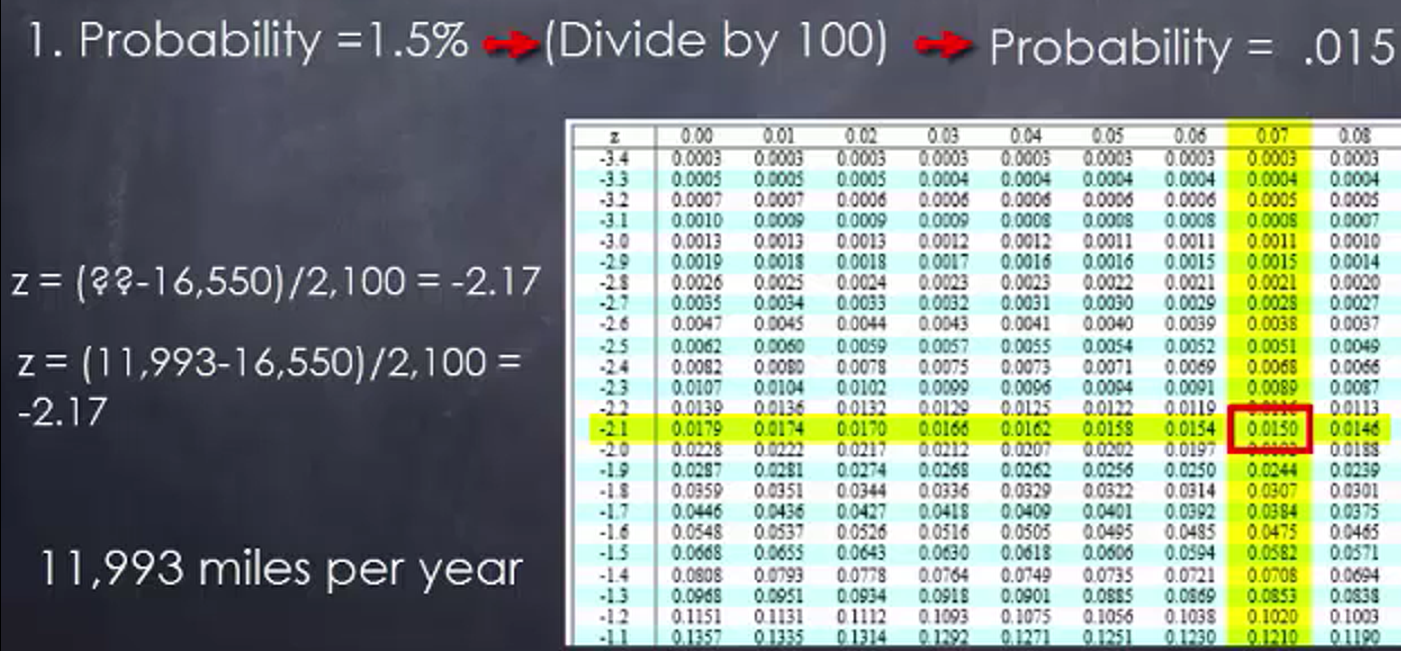You scan through the numbers until you find the value that’s equal to 0.015, which is going to be in the negative side of the z-table. Look at the columns and the rows, and you will find the value of 0.015 happens to have a z-value of -2.17. If you solve for that question mark, you’re going to wind up with a z-value of 11,993 miles per year. Only 1.5% of drivers drive fewer than that number of miles per year.

Suppose that you have a probability. Say you’re looking for the value of 69.5%. Once again, divide this value by 100 to arrive at a probability of 0.695, and scan the z-table to find that 0.695 value. It turns out that the z-value is equal to 0.51.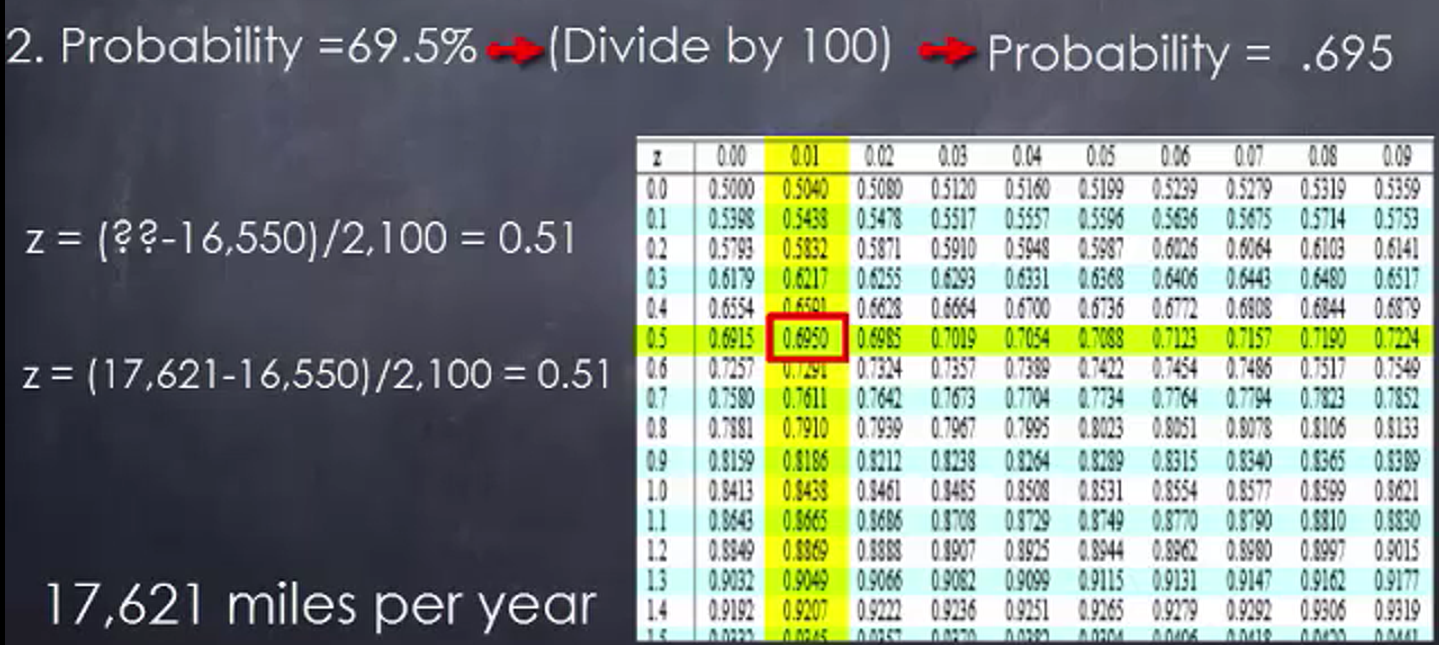To solve for your value, you look at whatever the value is minus 16,550. We take the difference and divide it by 2,100 to get 0.51. So if you do a little cross multiplication, you arrive at a value of 17,621 miles per year, in which case 69.5% of drivers would drive less than that.

summary
You reviewed how normal distributions and z-scores relate. This lead into z-tables. These are a way to look up more detailed information that a graph can give. You also saw how z-tables are used in several examples. It can be used to determine the likelihood of a range of values of a normally distributed variable occurring. You can also reverse it and look at the probability first. You saw how from there you can figure out what the z-score would be.

Source: This work is adapted from Sophia author Dan Laub.

Terms to Know
Z-Table

Table for looking up the area starting at z=0 for a positive z-score.

Rating